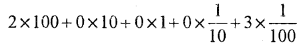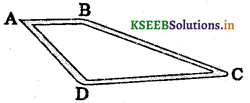# KSEEB Solutions for Class 7 Maths Chapter 2 Fractions haand Decimals Ex 2.5

Students can Download Class 7 Maths Chapter 2 Fractions and Decimals Ex 2.5 Questions and Answers, Notes Pdf, KSEEB Solutions for Class 7 Maths helps you to revise the complete Karnataka State Board Syllabus and to clear all their doubts, score well in final exams.

## Karnataka State Syllabus Class 7 Maths Chapter 2 Fractions and Decimals Ex 2.5

Question 1.
Which is greater?
i) 0.5 or 0.05
Solution:
0.5 > 0.05 (∵ 0.5 = 0.50)

ii) 0.7 or 0.5
Solution:
0.7 > 0.5

iii) 7 or 0.7
Solution:
7 > 0.7 (∵ 7 = 7.0)

iv) 1.37 or 1.49
Solution:
1.49 > 1.37

v) 2.03 or 2.30
Solution:
2.30 > 2.03

vi) 0.8 or 0.88
Solution:
0.88 > 0.8 (∵ 0.8 = 80)

Express 5/8 as a decimal.

Question 2.
Express as rupees using decimals :
i) 7 paise
Solution:
7 paise = Rs. 0.07

ii) 7 rupees 7 paise
Solution:
7 rupees 7 paise Rs. 7.07

iii) 77 rupees 77paise
Solution:
77 rupees 77 paise = ₹ 77.77

iv) 50 paise
Solution:
50 paise = 0.50

v) 235 paise
Solution:
235 paise = 2.35Question 3.
i) Express 5cm in metre and kilometre
Solution:
5 cm = 0.05m = 0.00005km.

ii) Express 35mm in cm, m and km
Solution:
35mm = 3.5cm = 0.000035km

Question 4.
Express in kg :
i) 200g
Solution:
200g = 0.200kg = 0.2 kg

ii) 3470g
Solution:
3470g = 3.470kg

iii) 4 kg 8 g
Solution:
4kg 8g = 4.008 kg

The fraction 3 11/16 to decimal is equal to 19.4375 when converted to a decimal.

Question 5.
Write the following decimal numbers in the expanded form :
i) 20.03
Solution:ii) 2.03
Solution:iii) 200.03
Solution:iv) 2.034
Solution:Question 6.
Write the place value of 2 in the following decimal numbers:
(i) 2.56 (ii) 21.37 (iii) 10.25 (iv) 9.42 (v) 63.352
Solution:
(i) 2.56 : Two is in ones place, its value is 2.
(ii) 21.37 : Two is in tens place, its value is 20
(iii) 10.25 : Two is in tenth place, its value is $$\frac{2}{10}$$
(iv) 9.42 : Two is in hundredths place, its value is $$\frac{2}{100}$$
(v) 63.352 : Two is in thousandths place, its value is $$\frac{2}{1000}$$

Question 7.
Dinesh went from place A to place B and from 12.7 km from C. Ayub went from place A to place D and from there to place C. D is 9.3 km from A and C is 11.8 km from D. Who travelles more and by how much?Solution:
Distance travelled from A to B = 7.5kms
Distance travelled from B to C = 12.7kms
Total distance travelled from A to B & B to C = 20.2 kms.
Ayub
Distance travelled from A to D = 9.3 kms
Distance travelled from D to C = 11.8 kms
Total distance travelled from A to D & D to C = 21.1 kms
∴ Ayub travelled more distance than Dinesh by = 21.1 – 20.2 = 0.9 kms.

Question 8.
Shyama bought 5 kg 300g apples and 3 kg 250 g mangoes. Sarala bought 4 kg 800 g oranges and 4 kg 150g bananas. Who bought more fruits?
Solution:
Shyama
Weight of Apples bought = 5.300kgs
Weight of Mangoes bought = 3.250kgs
Total weight of fruits = 8.550kgs
Sarala
Weight of oranges bought = 4.800kgs
Weight of Bananas bought = 4.150kgs
Total weight of fruits = 8.950kgs
8.950 > 8.550
∴ Sarala bought more fruits than Shyama.

Question 9.
How much less is 28 km than 42.6 km?
Solution:
(42.6 – 28)Km∴ 28 km is less than 42.6 km by 14.6 kms.